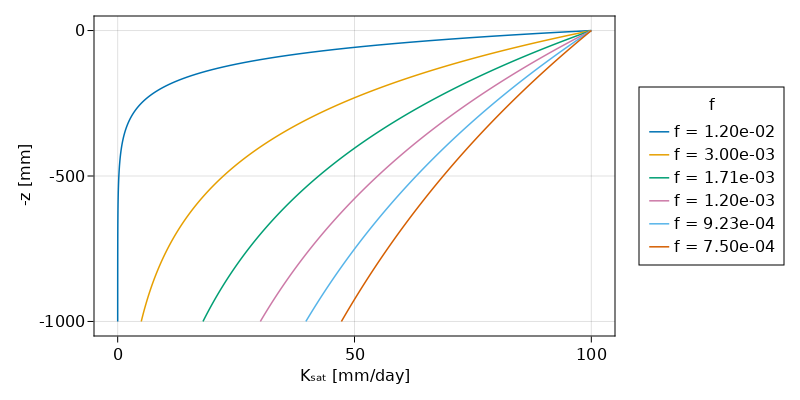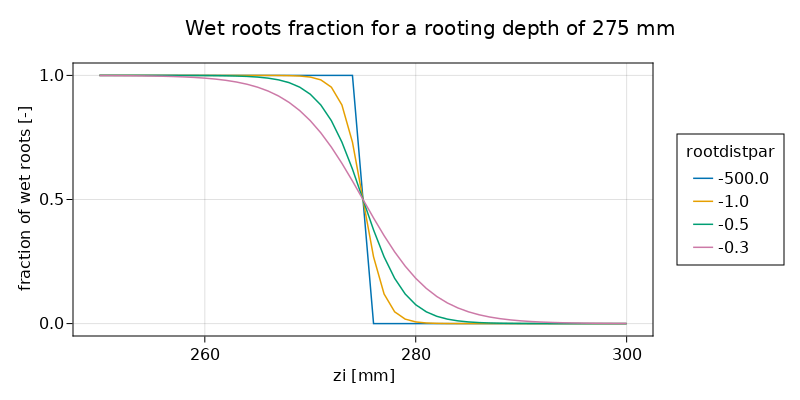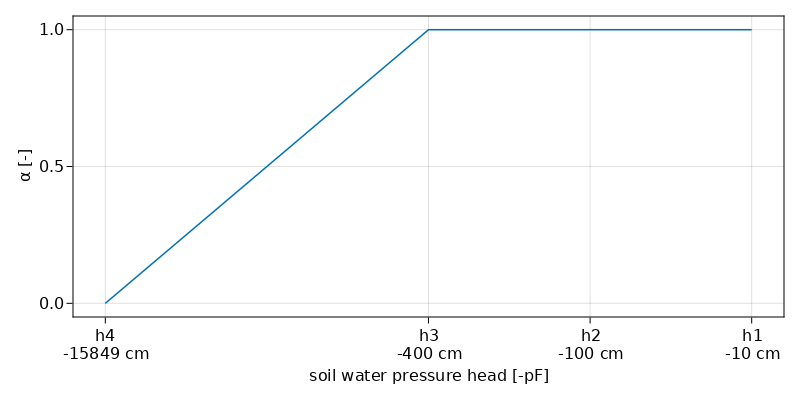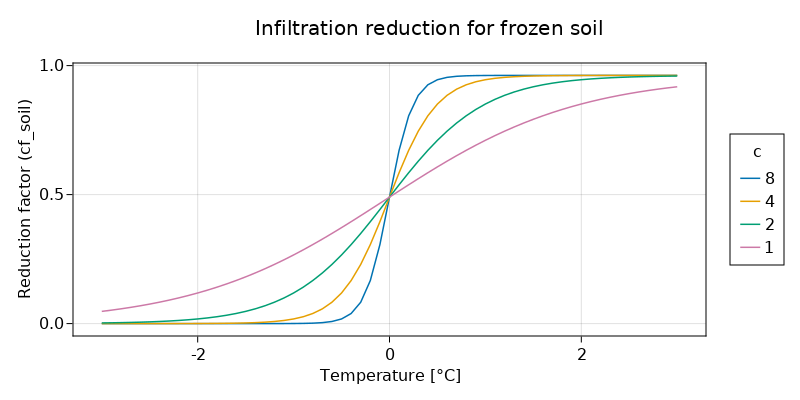SBM vertical concept

The SBM vertical concept has its roots in the Topog_SBM model but has had considerable changes over time. The main differences are:

• The unsaturated zone can be split-up in different layers
• The addition of evapotranspiration losses
• The addition of a capillary rise

The sections below describe the working of the SBM vertical concept in more detail.

Snow

Snow modelling is enabled by specifying the following in the TOML file:

[model]
snow = true

The snow model is described in Snow modelling

Glaciers

Glacier processes are described in Glacier modelling. Glacier modelling is enabled by specifying the following in the TOML file:

[model]
glacier = true

Soil

Infiltration

If the surface is (partly) saturated the throughfall and stemflow that falls onto the saturated area is added to the river runoff component (based on fraction rivers, riverfrac [-]) and to the overland runoff component (based on open water fraction (waterfrac [-] minus riverfrac [-]). Infiltration of the remaining water is determined as follows:

The soil infiltration capacity can be adjusted in case the soil is frozen, this is optional and can be set in the TOML file as follows:

[model]
soilinfreduction = true

The remaining storage capacity of the unsaturated store is determined. The infiltrating water is split in two parts, the part that falls on compacted areas and the part that falls on non-compacted areas. The maximum amount of water that can infiltrate in these areas is calculated by taking the minimum of the maximum infiltration rate (infiltcapsoil [mm t$^{-1}$] for non-compacted areas and infiltcappath [mm t$^{-1}$] for compacted areas) and the amount of water available for infiltration avail_forinfilt [mm t$^{-1}$]. The water that can actual infiltrate infiltsoilpath [mm t$^{-1}$] is calculated by taking the minimum of the total maximum infiltration rate (compacted and non-compacted areas) and the remaining storage capacity.

Infiltration excess occurs when the infiltration capacity is smaller then the throughfall and stemflow rate. This amount of water (infiltexcess [mm t$^{-1}$]) becomes overland flow (infiltration excess overland flow). Saturation excess occurs when the (upper) soil becomes saturated and water cannot infiltrate anymore. This amount of water excesswater [mm t$^{-1}$] becomes overland flow (saturation excess overland flow).

The SBM soil water accounting scheme

A detailed description of the Topog_SBM model has been given by Vertessy (1999). Briefly: the soil is considered as a bucket with a certain depth ($z_{t}$ [mm]), divided into a saturated store ($S$ [mm]) and an unsaturated store ($U$ [mm]). The top of the $S$ store forms a pseudo-water table at depth $z_{i}$ [mm] such that the value of $S$ at any time is given by:

$$$S=(z_{t}-z_{i})(\theta_{s}-\theta_{r})$$$

where $\theta_{s}$ [-] and $\theta_{r}$ [-] are the saturated and residual soil water contents, respectively.

The unsaturated store $U$ is subdivided into storage ($U_{s}$ [mm]) and deficit ($U_{d}$ [mm]):

$$$U_{d}=(\theta_{s}-\theta_{r})z_{i}-U\\ U_{s}=U-U_{d}$$$

The saturation deficit ($S_{d}$ [mm]) for the soil profile as a whole is defined as:

$$$S_{d}=(\theta_{s}-\theta_{r})z_{t}-S$$$

All infiltrating water that enters the $U$ store first. The unsaturated layer can be split-up in different layers, by providing the thickness [mm] of the layers in the TOML file. The following example specifies three layers (from top to bottom) of 100, 300 and 800 mm:

[model]
thicknesslayers = [100, 300, 800]

The code checks for each grid cell the specified layers against the soilthickness [mm], and adds or removes (partly) layer(s) based on the soilthickness.

Assuming a unit head gradient, the transfer of water ($st$ [mm t$^{-1}$]) from a $U$ [mm] store layer is controlled by the saturated hydraulic conductivity $K_{sat}$ [mm t$^{-1}$] at depth $z$ [mm] (bottom layer) or $z_{i}$ [mm], the effective saturation degree of the layer, and a Brooks-Corey power coefficient (parameter $c$) based on the pore size distribution index $\lambda$ (Brooks and Corey, 1964):

$$$st=K_{\mathit{sat}}\left(\frac{\theta-\theta_{r}}{\theta_{s}-\theta_{r}}\right)^{c}\\~\\ c=\frac{2+3\lambda}{\lambda}$$$

When the unsaturated layer is not split-up into different layers, it is possible to use the original Topog_SBM vertical transfer formulation, by specifying in the TOML file:

[model]
transfermethod = true

The transfer of water from the $U$ [mm] store to the $S$ [mm] store ($st$ [mm t$^{-1}$]) is in that case controlled by the saturated hydraulic conductivity $K_{sat}$ [mm t$^{-1}$] at depth $z_{i}$ [mm] and the ratio between $U$ [mm] and $S_{d}$ [mm]:

$$$st=K_{\mathit{sat}}\frac{U_{s}}{S_{d}}$$$

Saturated conductivity ($K_{sat}$ [mm t$^{-1}$]) declines with soil depth ($z$ [mm]) in the model according to:

$$$K_{sat}=K_{0}e^{(-fz)}$$$

where $K_{0}$ [mm t$^{-1}$] is the saturated conductivity at the soil surface and $f$ is a scaling parameter [mm$^{-1}$].

The plot below shows the relation between soil depth $z$ and saturated hydraulic conductivity $K_{sat}$ for different values of $f$.Transpiration and soil evaporation

The potential evaporation left over after interception and open water evaporation (rivers and water bodies) is split in potential soil evaporation and potential transpiration based on the canopy gap fraction (assumed to be identical to the amount of bare soil).

For the case of one single soil layer (the SBM soil column is not split-up into different layers), soil evaporation [mm t$^{-1}$] is scaled according to (as shown by the following code block (i refers to the index of the vector that contains all active cells within the spatial model domain)):

soilevapunsat = potsoilevap * min(1.0, saturationdeficit / sbm.soilwatercapacity[i])

As such, evaporation will be potential if the soil is fully wetted and it decreases linear with increasing soil moisture deficit.

For more than one soil layer, soil evaporation is only provided from the upper soil layer (often 100 mm) and soil evaporation is split in evaporation from the unsaturated store and evaporation from the saturated store. First water is evaporated water from the unsaturated store. Then the remaining potential soil evaporation can be used for evaporation from the saturated store. This is only possible, when the water table is present in the upper soil layer (very wet conditions). Both the evaporation from the unsaturated store and the evaporation from the saturated store are limited by the minimum of the remaining potential soil evaporation and the available water in the unsaturated/saturated zone of the upper soil layer. Also for multiple soil layers, the evaporation (both unsaturated and saturated) decreases linearly with decreasing water availability.

The original Topog_SBM model does not include transpiration or a notion of capillary rise. In SBM transpiration is first taken from the $S$ [mm] store if the roots reach the water table $z_{i}$ [mm]. If the $S$ [mm] store cannot satisfy the demand the $U$ [mm] store is used next. First the fraction of wet roots (wetroots [-]) is determined (going from 1 to 0) using a sigmoid function as follows:

$$$wetroots = 1.0/(1.0 + e^{-rootdistpar (zi - rootingdepth)})$$$

Below a plot showing the fraction of wet roots for different values of rootdistpar [-] for a rooting depth of 275 mm.Here the sharpness parameter rootdistpar [-] (by default a large negative value, -500.0) determines if there is a stepwise output or a more gradual output (default is stepwise). zi [mm] is the level of the water table in the grid cell below the surface, rootingdepth [mm] is the maximum depth of the roots below the surface. For all values of zi smaller that rootingdepth a value of 1 is returned if they are equal a value of 0.5 is returned if zi is larger than the rootingdepth a value of 0 is returned. The returned wetroots [-] fraction is multiplied by the potential evaporation (and limited by the available water in saturated zone) to get the transpiration from the saturated part of the soil, as shown by the following code block (i refers to the index of the vector that contains all active cells within the spatial model domain):

# transpiration from saturated store
wetroots = scurve(sbm.zi[i], rootingdepth, 1.0, sbm.rootdistpar[i])
actevapsat = min(pottrans * wetroots, satwaterdepth)
satwaterdepth = satwaterdepth - actevapsat
restpottrans = pottrans - actevapsat

Next the remaining potential evaporation is used to extract water from the unsaturated store. The fraction of roots (availcap [-]) that cover the unsaturated zone for each soil layer is used to calculate the potential root water extraction rate (maxextr [mm t$^{-1}$]). When whole_ust_available is set to true in the TOML file as follows, almost the complete unsaturated storage (99%) is available for transpiration, independent of the rootingdepth:

[model]
whole_ust_available = true

Below the code snippet from the Wflow.acttransp_unsat_sbm function that calculates the potential root water extraction rate maxextr [mm t$^{-1}$], as shown by the following code block:

if ust # whole_ust_available = true
availcap = ustorelayerdepth * 0.99
else
if usl > 0.0
availcap = min(1.0, max(0.0, (rootingdepth - sumlayer) / usl))
else
availcap = 0.0
end
end
maxextr = availcap * ustorelayerdepth

where ustorelayerdepth [mm] is the amount of water in the unsaturated layer, sumlayer [mm] is the upper boundary (depth) of the unsaturated zone layer, and usl [mm] is the thickness of the unsaturated zone layer and availcap and maxextr as previously defined.

Next, the Feddes root water uptake reduction model (Feddes et al., 1978) is used to calculate a reduction coefficient as a function of soil water pressure. Soil water pressure is calculated following Brooks and Corey (1964):

$$$\frac{(\theta-\theta_r)}{(\theta_s-\theta_r)} = \Bigg\lbrace{\left(\frac{h_b}{h}\right)^{\lambda}, h > h_b \atop 1 , h \leq h_b}$$$

where $h$ is the pressure head [cm], $h_b$ is the air entry pressure head [cm], and $\theta$, $\theta_s$, $\theta_r$ and $\lambda$ as previously defined.

Feddes (1978) described a transpiration reduction-curve for the reduction coefficient $\alpha$, as a function of $h$. The plot below shows the reduction curve as implemented for the SBM concept, with fixed values for h2, h3 and h4, while h1 has a default value of -10 cm and can be provided as part of the static input. Root water uptake is zero when the soil water pressure head is below the wilting point h4, and reduced in the dry range between h4 and the critical head value h3. Optimal conditions for root water uptake exist when the soil water pressure head is above the critical head value h3. Note that in the original transpiration reduction-curve of Feddes (1978) root water uptake above h1 is set to zero (oxygen deficit) and between h1 and h2 root water uptake is limited. The assumption that very wet conditions do not affect root water uptake too much is probably generally applicable to natural vegetation, however for crops this assumption is not valid. This could be improved in the Wflow code by applying the reduction to crops only.Below, the function used in SBM, that calculates actual transpiration from the unsaturated zone layer(s).

Wflow.acttransp_unsat_sbmFunction
acttransp_unsat_sbm(rootingdepth, ustorelayerdepth, sumlayer, restpotevap, sum_actevapustore, c, usl, θₛ, θᵣ, hb, ust::Bool = false)

Compute actual transpiration for unsaturated zone. If ust is true, the whole unsaturated store is available for transpiration.

Arguments

• rootingdepth
• ustorelayerdepth
• sumlayer (depth (z) of upper boundary unsaturated layer)
• restpotevap (remaining evaporation)
• sum_actevapustore (cumulative actual transpiration (more than one unsaturated layers))
• c (Brooks-Corey coefficient)
• usl (thickness of unsaturated zone)
• θₛ
• θᵣ
• hb (air entry pressure)
• ust

Output

• ustorelayerdepth
• sum_actevapustore
• restpotevap
source

The actual capillary rise actcapflux [mm t$^{-1}$] is determined using the following approach: first the saturated hydraulic conductivty ksat [mm t$^{-1}$] is determined at the water table $z_{i}$; next a potential capillary rise maxcapflux [mm t$^{-1}$] is determined from the minimum of ksat, actual transpiration actevapustore [mm t$^{-1}$] taken from the $U$ store, available water in the $S$ store (satwaterdepth [mm]) and the deficit of the $U$ store (ustorecapacity [mm]), as shown by the following code block:

maxcapflux = max(0.0, min(ksat, actevapustore, ustorecapacity, satwaterdepth))

Then the potential rise maxcapflux is scaled using the water table depth zi, a maximum water depth cap_hmax [mm] beyond which capillary rise ceases and a coefficient cap_n [-], as follows in the code block below (i refers to the index of the vector that contains all active cells within the spatial model domain):

if sbm.zi[i] > rootingdepth
capflux =
maxcapflux * pow(
1.0 - min(sbm.zi[i], sbm.cap_hmax[i]) / (sbm.cap_hmax[i]),
sbm.cap_n[i],
)
else
capflux = 0.0
end

If the roots reach the water table (rootingdepth $\ge$ sbm.zi), capflux is set to zero.

Finally, the capillary rise capflux is limited by the unsaturated store deficit (one or multiple layers), calculated as follows in the code block below (i refers to the index of the vector that contains all active cells within the spatial model domain, and k refers to the layer position):

usl[k] * (sbm.θₛ[i] - sbm.θᵣ[i]) - usld[k]

where usl [mm] is the unsaturated layer thickness, usld is the ustorelayerdepth [mm] (amount of water in the unsaturated layer), and $\theta_{s}$ and $\theta_{r}$ as previously defined.

The calculation of the actual capillary rise actcapflux is as follows in the code block below (i refers to the index of the vector that contains all active cells within the spatial model domain, and k refers to the layer position):

actcapflux = 0.0
netcapflux = capflux
for k = n_usl:-1:1
min(netcapflux, max(usl[k] * (sbm.θₛ[i] - sbm.θᵣ[i]) - usld[k], 0.0))
usld = setindex(usld, usld[k] + toadd, k)
end

In case of multiple unsaturated layers (n_usl $>$ 1), the calculation of the actual capillary rise starts at the lowest unsaturated layer while keeping track of the remaining capillary rise netcapflux [mm t$^{-1}$].

Leakage

If the maxleakage [mm/day] input model parameter is set > 0, water is lost from the saturated zone and runs out of the model.

Soil temperature

The near surface soil temperature is modelled using a simple equation (Wigmosta et al., 2009):

$$$T_s^{t} = T_s^{t-1} + w (T_a - T_s^{t-1})$$$

where $T_s^{t}$ [$\degree$C] is the near-surface soil temperature at time $t$, $T_a$ [$\degree$C] is air temperature and $w$ [-] is a weighting coefficient determined through calibration (default is 0.1125 for daily timesteps).

A reduction factor (cf_soil [-], default is 0.038) is applied to the maximum infiltration rate (infiltcapsoil and infiltcappath), when the following model settings are specified in the TOML file:

[model]
soilinfreduction = true
snow = true

A S-curve (see plot below) is used to make a smooth transition (a c-factor ($c$) of 8.0 is used):

$$$b = \frac{1.0}{(1.0 - cf\_soil)}\\~\\ soilinfredu = \frac{1.0}{b + exp(-c (T_s - a))} + cf\_soil\\~\\ a = 0.0\\ c = 8.0$$$• Brooks, R. H., and Corey, A. T., 1964, Hydraulic properties of porous media, Hydrology Papers 3, Colorado State University, Fort Collins, 27 p.
• Feddes, R.A., Kowalik, P.J. and Zaradny, H., 1978, Simulation of field water use and crop yield, Pudoc, Wageningen, Simulation Monographs.
• Vertessy, R., and Elsenbeer, H., 1999, Distributed modeling of storm ﬂow generation in an amazonian rain forest catchment: effects of model parameterization, Water Resour. Res., 35, 2173–2187. doi: 10.1029/1999WR9000511257.
• Wigmosta, M. S., Lane, L. J., Tagestad, J. D., and Coleman A. M., 2009, Hydrologic and erosion models to assess land use and management practices affecting soil erosion, J. Hydrol. Eng., 14, 27-41.